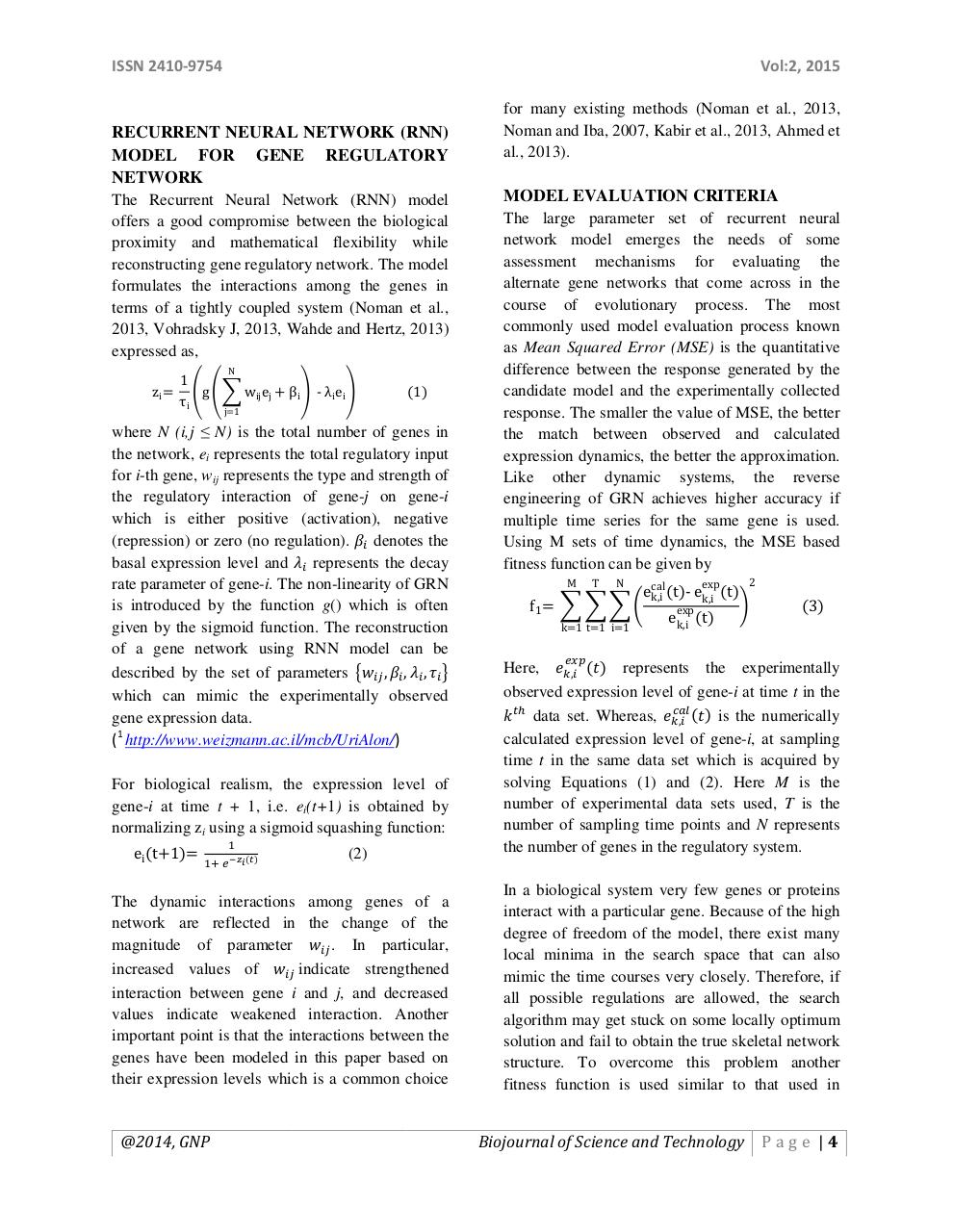# m140007.pdfPage 1 2 3 4 5 6 7 8 9 10 11

#### Text preview

ISSN 2410-9754

Vol:2, 2015

RECURRENT NEURAL NETWORK (RNN)
MODEL FOR GENE REGULATORY
NETWORK
The Recurrent Neural Network (RNN) model
offers a good compromise between the biological
proximity and mathematical flexibility while
reconstructing gene regulatory network. The model
formulates the interactions among the genes in
terms of a tightly coupled system (Noman et al.,
2013, Vohradsky J, 2013, Wahde and Hertz, 2013)
expressed as,
zi =

N

1
ቌg ቌ෍ wij ej + βi ቍ - λi ei ቍ
τi
j=1

(1)

where N (i,j ≤ N) is the total number of genes in
the network, ei represents the total regulatory input
for i-th gene, wij represents the type and strength of
the regulatory interaction of gene-j on gene-i
which is either positive (activation), negative
(repression) or zero (no regulation). ߚ௜ denotes the
basal expression level and ߣ௜ represents the decay
rate parameter of gene-i. The non-linearity of GRN
is introduced by the function g() which is often
given by the sigmoid function. The reconstruction
of a gene network using RNN model can be
described by the set of parameters ൛‫ݓ‬௜௝ , ߚ௜ , ߣ௜ , ߬௜ ൟ
which can mimic the experimentally observed
gene expression data.
(1 http://www.weizmann.ac.il/mcb/UriAlon/)
For biological realism, the expression level of
gene-i at time t + 1, i.e. ei(t+1) is obtained by
normalizing zi using a sigmoid squashing function:
ei (t+1)=

ଵା ௘ ష೥೔ (೟)

(2)

The dynamic interactions among genes of a
network are reflected in the change of the
magnitude of parameter ‫ݓ‬௜௝ . In particular,
increased values of ‫ݓ‬௜௝ indicate strengthened
interaction between gene i and j, and decreased
values indicate weakened interaction. Another
important point is that the interactions between the
genes have been modeled in this paper based on
their expression levels which is a common choice

@2014, GNP

for many existing methods (Noman et al., 2013,
Noman and Iba, 2007, Kabir et al., 2013, Ahmed et
al., 2013).

MODEL EVALUATION CRITERIA
The large parameter set of recurrent neural
network model emerges the needs of some
assessment mechanisms for evaluating the
alternate gene networks that come across in the
course of evolutionary process. The most
commonly used model evaluation process known
as Mean Squared Error (MSE) is the quantitative
difference between the response generated by the
candidate model and the experimentally collected
response. The smaller the value of MSE, the better
the match between observed and calculated
expression dynamics, the better the approximation.
Like other dynamic systems, the reverse
engineering of GRN achieves higher accuracy if
multiple time series for the same gene is used.
Using M sets of time dynamics, the MSE based
fitness function can be given by
2

ecal
k,i (t)- ek,i (t)
f1 = ෍ ෍ ෍ ቆ

exp
ek,i (t)
M

T

N

k=1 t=1 i=1

exp

(3)

Here, ݁௞,௜ (‫ )ݐ‬represents the experimentally
observed expression level of gene-i at time t in the
௖௔௟
(‫ )ݐ‬is the numerically
݇ ௧௛ data set. Whereas, ݁௞,௜
calculated expression level of gene-i, at sampling
time t in the same data set which is acquired by
solving Equations (1) and (2). Here M is the
number of experimental data sets used, T is the
number of sampling time points and N represents
the number of genes in the regulatory system.
௘௫௣

In a biological system very few genes or proteins
interact with a particular gene. Because of the high
degree of freedom of the model, there exist many
local minima in the search space that can also
mimic the time courses very closely. Therefore, if
all possible regulations are allowed, the search
algorithm may get stuck on some locally optimum
solution and fail to obtain the true skeletal network
structure. To overcome this problem another
fitness function is used similar to that used in

Biojournal of Science and Technology P a g e | 4